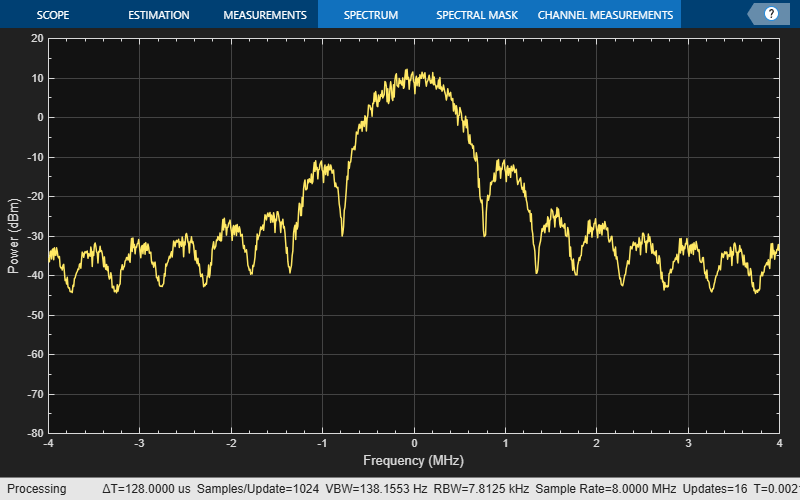# bleWaveformGenerator

Waveform generator for BLE PHY

## Syntax

``waveform = bleWaveformGenerator(message)``
``waveform = bleWaveformGenerator(message,Name,Value)``

## Description

example

````waveform = bleWaveformGenerator(message)` generates `waveform`, a time-domain Bluetooth low energy (BLE) physical layer (PHY) waveform by using the input information bits `message`.```

example

````waveform = bleWaveformGenerator(message,Name,Value)` also specifies options using one or more name-value pair arguments. For example, `'Mode','LE2M'` specifies the generating mode value of the desired BLE waveform.```

## Examples

collapse all

Create an input message column vector of length 2056 containing random binary values. Set the symbol rate to default value.

```message = randi([0 1],2056,1); symbolRate = 1e6;```

Generate the BLE waveform.

`waveform = bleWaveformGenerator(message);`

Create a `dsp.SpectrumAnalyzer` System object to display the frequency spectrum of the generated BLE waveform. Set the sample rate of the frequency spectrum.

```scope = dsp.SpectrumAnalyzer; scope.SampleRate = 8*symbolRate;```

Plot the BLE waveform.

`scope(waveform);`Create an input message column vector of length 640 containing random binary values.

`message = randi([0 1],640,1);`

Specify the values of generating mode, channel index, samples per symbol and access address. Set symbol rate to default value.

```phyMode = 'LE125K'; chanIdx = 2; sps = 4; accAdd = [1 1 1 1 0 1 0 0 1 1 0 1 0 0 1 0 0 1 1 0 1 1 1 0 1 ... 0 1 0 1 1 0 0].'; symbolRate = 1e6;```

Create a `dsp.SpectrumAnalyzer` System object to display the frequency spectrum of the generated BLE waveform. Set the sample rate of the frequency spectrum.

```scope = dsp.SpectrumAnalyzer; scope.SampleRate = sps*symbolRate;```

Generate the BLE waveform using specified name-value pair arguments. Plot the BLE waveform.

```waveform = bleWaveformGenerator(message,'Mode',phyMode, ... 'SamplesPerSymbol',sps,'ChannelIndex',chanIdx,'AccessAddress',accAdd); scope(waveform);```## Input Arguments

collapse all

Input message bits, specified as a binary-valued column vector of numerical or logical values. This message contains the protocol data unit (PDU) and cyclic redundancy check (CRC) data. The maximum length of this value is 2080 bits.

Data Types: `double` | `logical` | `single` | `int`

### Name-Value Pair Arguments

Specify optional comma-separated pairs of `Name,Value` arguments. `Name` is the argument name and `Value` is the corresponding value. `Name` must appear inside quotes. You can specify several name and value pair arguments in any order as `Name1,Value1,...,NameN,ValueN`.

Example: `bleWaveformGenerator(message,'Mode','LE2M','ChannelIndex',36)`

Generating mode, specified as the comma-separated pair consisting of `'Mode'` and `'LE1M'`, `'LE2M'`, `'LE500K'`, or `'LE125K'`. This value indicates the type of BLE waveform.

Data Types: `string` | `char`

Channel index, specified as the comma-separated pair consisting of `'ChannelIndex'`and an integer in the range [0, 39]. For data channels, this value must be in the range [0, 36]. For advertising channels, this value must be in the range [37, 39]. This value is used by the data-whitening block to randomize the bits.

Data Types: `single` | `double`

Samples per symbol, specified as the comma-separated pair consisting of `'SamplesPerSymbol'` and a positive integer. This value is used for the Gaussian frequency-shift keying (GFSK) modulation.

Data Types: `single` | `double`

Access address, specified as the comma-separated pair consisting of `'AccessAddress'` and a 32-bit column vector of numerical or logical values.

Data Types: `logical` | `single` | `double` | `int`

## Output Arguments

collapse all

Output time-domain waveform, returned as a complex-valued column vector of size Ns-by-1, where Ns represents the number of time-domain samples. The `waveform` is generated in the form of complex in-phase quadrature (IQ) samples.

 Bluetooth Technology Website. “Bluetooth Technology Website | The Official Website of Bluetooth Technology.” Accessed November 22, 2019. https://www.bluetooth.com/.

 Bluetooth Special Interest Group (SIG). "Bluetooth Core Specification." Version 5.1. https://www.bluetooth.com/.